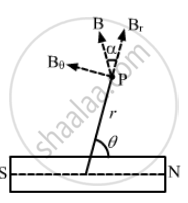Department of Pre-University Education, KarnatakaPUC Karnataka Science Class 12
Advertisement Remove all ads

# Show that the Magnetic Field at a Point Due to a Magnetic Dipole is Perpendicular to the Magnetic Axis If the Line Joining the Point with the Centre of the Dipole Makes an Angle - Physics

Sum

Show that the magnetic field at a point due to a magnetic dipole is perpendicular to the magnetic axis if the line joining the point with the centre of the dipole makes an angle of tan^-1(sqrt 2) with the magnetic axis

Advertisement Remove all ads

#### Solution

Given :

Angle made by observation point P with the axis of the dipole, θ = tan^-1 (sqrt 2)

⇒ tan θ = sqrt 2

⇒ 2 = tan^2 θ

⇒ tan θ = cot θ

⇒ tan θ /2 = cot θ         .....(1)

we know ,

tan θ /2 = tan α      ....(2)On comparing (1) and (2), we get

tan α = cot θ

⇒ tan α = tan (90 - θ)

⇒ α = 90 - θ

⇒ θ + α = 90^circ

Hence, the magnetic field due to the dipole is perpendicular to the magnetic axis.

Is there an error in this question or solution?
Advertisement Remove all ads

#### APPEARS IN

HC Verma Class 11, Class 12 Concepts of Physics Vol. 2
Chapter 14 Permanent Magnets
Q 6 | Page 277
Advertisement Remove all ads

#### Video TutorialsVIEW ALL 

Advertisement Remove all ads
Share
Notifications

View all notifications

Forgot password?